﻿ 基于经验模态分解理论的去噪方法研究 The Research of Denoising Method Based on Empirical Mode Decomposition Theory

Vol.06 No.07(2017), Article ID:22548,11 pages
10.12677/AAM.2017.67106

The Research of Denoising Method Based on Empirical Mode Decomposition Theory

Shuwen Niu

School of Mathematics and Physics, China University of Geosciences, Wuhan Hubei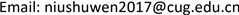Received: Oct. 9th, 2017; accepted: Oct. 23rd, 2017; published: Oct. 31st, 2017ABSTRACT

Based on the basic idea of empirical mode decomposition method, this paper presents a new filtering method by using the significant differences between the noise curvature curve and the discrete Frechet distance of the signal curvature curve. Using this method to analyze the simulation data, the results show that the new filtering method is an effective denoising method. Compared with the traditional empirical mode decomposition (EMD) method, this method can denoise the signal and have high signal to noise ratio. This method can effectively reduce the influence of noise on the signal, then extract useful information from the noisy signal.

Keywords:Empirical Mode Decomposition, Curvature, Discrete Frechet Distance, Signal Denoising1. 引言

2. EMD及其改进方法

2.1. EMD以及相关方法

EMD方法依据输入信号自身的特点，自适应地将信号分解成若干个本征模态函数(intrinsic mode function, IMF)之和。每个IMF分量必须满足以下两个条件：1) 在整个时间范围内，局部极值点和过零点的数目必须相等，或最多相差一个；2) 在任意时刻点，局部最大值的包络(上包络线)和局部最小值的包络(下包络线)的平均值必须为零  。

EMD方法实际上是一种循环迭代算法，该算法的具体过程如下：

1) 分别找出原数据序列 $X\left(t\right)$ 所有的极大值点和极小值点，并用三次样条插值函数拟合形成原数据的上包络线和下包络线。

2) 计算上包络线和下包络线的均值，记作 ${m}_{1}\left(t\right)$ ，用原数据序列 $X\left(t\right)$ 减去该均值，即可得到一个去掉低频的新数据序列 ${h}_{1}\left(t\right)$

$X\left(t\right)-{m}_{1}\left(t\right)={h}_{1}\left(t\right)$

3) 由原数据序列减去均值后得到的新数据序列 ${h}_{1}\left(t\right)$ ，若满足IMF的条件，则作为第一个IMF分量序列，否则，需要对它重复进行上述处理过程。重复进行上述处理过程k次后，直到 ${h}_{1}\left(t\right)$ 符合IMF的定义要求，所得到的均值趋于零为止，这样就得到了第1个IMF分量 ${c}_{1}\left(t\right)$ ，它代表信号 $X\left(t\right)$ 中最高频率的分量：

$\begin{array}{l}{h}_{1\left(k-1\right)}\left(t\right)-{m}_{1k}\left(t\right)={h}_{1k}\left(t\right)\\ \text{ }{c}_{1}\left(t\right)={h}_{1k}\left(t\right)\end{array}$

4) 将 ${c}_{1}\left(t\right)$$X\left(t\right)$ 中分离出来，即得到一个去掉高频分量的差值信号 ${r}_{1}\left(t\right)$ ，即有：

${r}_{1}\left(t\right)=X\left(t\right)-{c}_{1}\left(t\right)$

${r}_{1}\left(t\right)$ 作为原始数据，重复步骤(1)~(3)，即可得到第二个IMF分量 ${c}_{2}\left(t\right)$ ，重复n次，得到n个IMF分量，则有：

$\left\{\begin{array}{l}{r}_{1}\left(t\right)-{c}_{2}\left(t\right)={r}_{2}\left(t\right)\\ \text{ }\text{ }\text{ }\text{ }\text{ }\text{ }\text{ }\text{ }\text{ }\text{ }\text{ }\text{ }\text{ }\text{ }\text{ }\text{ }\text{ }\text{ }\text{ }\text{ }\text{ }\text{ }\text{ }\text{ }\text{ }\text{ }\text{ }\text{ }\text{ }\text{ }\text{ }\text{ }\text{ }\text{ }\text{ }\text{ }\text{ }\text{ }\text{ }\text{ }⋮\\ {r}_{n-1}\left(t\right)-{c}_{n}\left(t\right)={r}_{n}\left(t\right)\end{array}$

${r}_{n}\left(t\right)$ 满足给定的终止条件(通常使 ${r}_{n}\left(t\right)$ 成为一个单调函数)时，循环结束。原始信号 $X\left(t\right)$ 经过n次分解后可以写成以下表达式：

$X\left(t\right)=\sum _{j=1}^{n}{c}_{j}\left(t\right)+{r}_{n}\left(t\right)$

EEMD方法、CEEMD方法以及CEEMDAN方法如图1所示。

2.2. ICEEMDAN方法

1) 对信号 ${X}^{\left(i\right)}\left(t\right)\text{ }=X\left(t\right)+\text{ }\text{ }\text{ }{\beta }_{0}{E}_{1}\left({w}^{\left(i\right)}\left(t\right)\right)$ 进行I次EMD分解，计算出局部均值，得到第一个剩余分量，即 ${r}_{1}\left(t\right)=〈M\left({X}^{\left(i\right)}\left(t\right)\right)〉$ 。其中， ${\beta }_{0}={\epsilon }_{0}std\left(X\left(t\right)\right)/std\left({E}_{1}\left({w}^{\left(i\right)}\left(t\right)\right)\right)$

2) 当 $k=1$ 时，计算出第一个固有模态分量为 $\overline{IM{F}_{1}}\left(t\right)=X\left(t\right)-{r}_{1}\left(t\right)$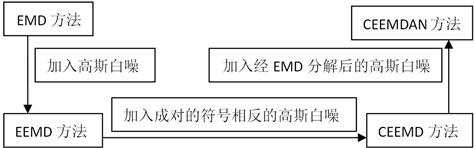Figure 1. EMD and related methods

3) 对信号 ${r}_{1}\left(t\right)+{\beta }_{1}{E}_{2}\left({w}^{\left(i\right)}\left(t\right)\right)$ 进行I次EMD分解，计算出局部均值，得到第二个剩余分量，即 ${r}_{2}\left(t\right)=〈M\left({r}_{1}\left(t\right)+{\beta }_{1}{E}_{2}\left({w}^{\left(i\right)}\left(t\right)\right)\right)〉$ 。则第二个固有模态分量为

$\overline{IM{F}_{2}}\left(t\right)={r}_{1}\left(t\right)-{r}_{2}\left(t\right)={r}_{1}\left(t\right)-〈M\left({r}_{1}\left(t\right)+{\beta }_{1}{E}_{2}\left({w}^{\left(i\right)}\left(t\right)\right)\right)〉$

4) 计算出第k个剩余分量和第k个固有模态分量 $\left(k=3,\cdots ,K\right)$

${r}_{k}\left(t\right)=〈M\left({r}_{k-1}\left(t\right)+{\beta }_{k-1}{E}_{k}\left({w}^{\left(i\right)}\left(t\right)\right)\right)〉$

$\overline{IM{F}_{k}}\left(t\right)={r}_{k-1}\left(t\right)-{r}_{k}\left(t\right)$

5) 重复步骤(4)，计算出下一个k。直到获得的残余分量不能被EMD进一步分解，即残余分量为一个单调函数为止。

3. 基于曲率–离散Frechet距离的重构分量选择

3.1. 曲线的曲率

$k=|\frac{{y}^{″}}{{\left(1+{{y}^{\prime }}^{2}\right)}^{\frac{3}{2}}}|$ (1)

3.2. Frechet距离

Frechet距离(Frechet Distance, FD)  是法国数学家Maurice Rene Frechet在1906年提出的一种空间路径的相似性描述，指利用两个目标的路径以及两条曲线上所有离散点的距离，量化两条曲线的相似度。这种方法直观，而且与Hausdorff距离等其他相似度量化方法相比，其可以更好地刻画折线曲线的相似度。设定t是时间点，该时刻，曲线a上的采样点为 $A\left(\alpha \left(t\right)\right)$ ，曲线B上采样点为 $B\left(\beta \left(t\right)\right)$ 。如果使用欧氏距离，则容易定义 $d\left(A\left(\alpha \left(t\right)\right),B\left(\beta \left(t\right)\right)\right)=‖A\left(\alpha \left(t\right)\right)-B\left(\beta \left(t\right)\right)‖$ 。在每次采样中t离散的遍历区间 $\left[0,1\right]$ ，得到该种采样下的最大距离 $\underset{t\in \left[0,1\right]}{\mathrm{max}}\left\{d\left(A\left(\alpha \left(t\right)\right),B\left(\beta \left(t\right)\right)\right)\right\}$ 。Frechet距离就是使该最大距离最小化的采样方式下的值。在离散方式下，我们不可能得到真实的Frechet距离，而可以无限的趋近。离散Frechet距离 $F\left(A,B\right)$ 定义为：

$\begin{array}{l}F\left(A,B\right)=\underset{n\to \infty ,\mathrm{max}|{t}_{k}-{t}_{k+1}|\to 0}{\mathrm{lim}}{\stackrel{˜}{F}}^{n}\left(A,B\right)\\ \text{ }\text{ }\text{ }\text{ }\text{ }\text{ }\text{ }\text{ }\text{ }\text{ }\text{ }\text{ }\text{ }\text{ }\text{ }\text{ }\text{ }\text{ }\text{ }\text{ }\text{ }\text{ }\text{ }\text{ }\text{ }\text{ }\text{ }\text{ }\text{ }\text{ }\text{ }\text{ }\text{ }\text{ }\text{ }\text{ }\text{ }\text{ }\text{ }\text{ }\text{ }\text{ }=\underset{n\to \infty ,\mathrm{max}|{t}_{k}-{t}_{k+1}|\to 0}{\mathrm{lim}}\underset{\alpha ,\beta \text{ }\text{ }\text{ }t\in \left[0,1\right]}{\mathrm{inf}\text{ }\mathrm{max}}\left\{{d}^{n}\left(A\left(\alpha \left(t\right)\right),B\left(\beta \left(t\right)\right)\right)\right\}\end{array}$ (2)

3.3. 重构分量的选择

$y\left(t\right)=\sum _{i=1}^{k-1}IM{F}_{i}+\sum _{i=k}^{n}IM{F}_{i}+{r}_{n}\left(t\right)$ (3)

1) 运用EMD、EEMD、CEEMD、CEEMDAN和ICEEMDAN 5种方法分解信号 $y\left(t\right)$ ，得到本征模态分量。

2) 分别计算出信号 $y\left(t\right)$ 和每个本征模态分量的曲率。

3) 计算信号 $y\left(t\right)$ 的曲率和每个本征模态分量曲率之间的离散Frechet距离。

4) 取离散Frechet距离的最小值所对应的本征模态分量的序号为k值。

5) 信号 $y\left(t\right)$ 可以重构为：

$y\left(t\right)=\sum _{i=k}^{n}IM{F}_{i}+{r}_{n}\left(t\right)$ (4)

4. 仿真实验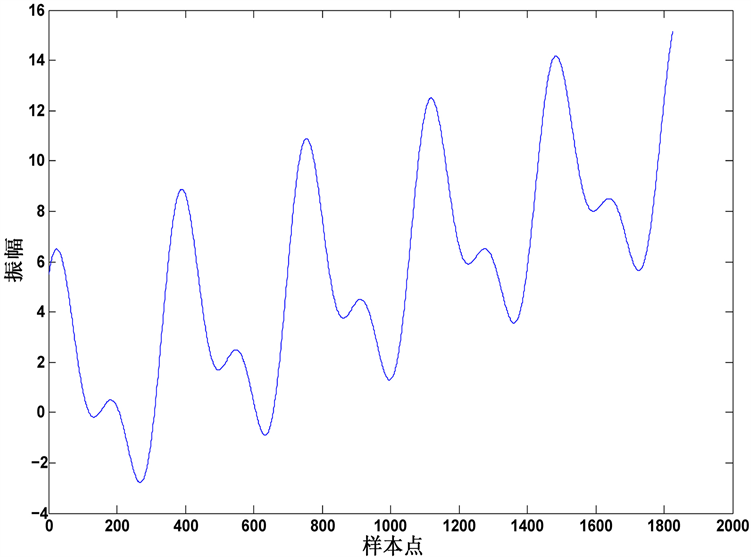Figure 2. Signal and curvature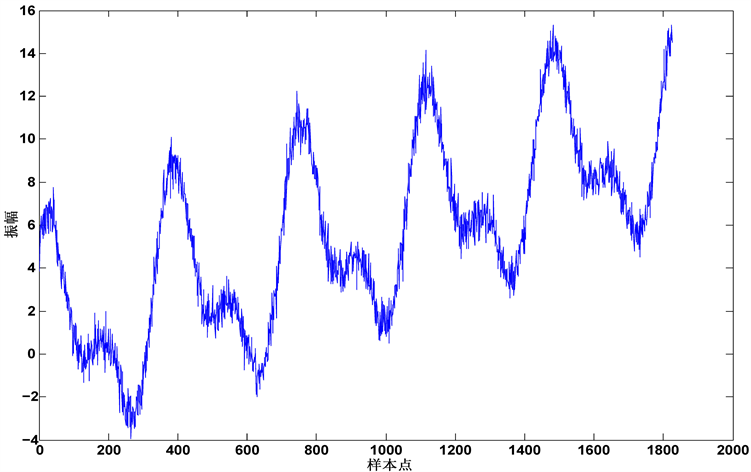Figure 3. Noisy signal waveform

$\begin{array}{l}y\left({t}_{i}\right)=a+b{t}_{i}+d\mathrm{cos}\left(2\text{π}{t}_{i}\right)+e\mathrm{sin}\left(2\text{π}{t}_{i}\right)\text{ }\text{ }\text{ }\text{ }+c\left({t}_{i}\right)\mathrm{cos}\left(2\text{π}{t}_{i}\right)+c\left({t}_{i}\right)\mathrm{sin}\left(2\text{π}{t}_{i}\right)+f\mathrm{cos}\left(4\text{π}{t}_{i}\right)+g\mathrm{sin}\left(4\text{π}{t}_{i}\right)\\ \text{ }\text{ }\text{ }\text{ }\text{ }\text{ }\text{ }\text{ }\text{ }\text{ }\text{ }\text{ }\text{ }\text{ }\text{ }\text{ }\text{ }\text{ }\text{ }\text{ }\text{ }\text{ }\text{ }\text{ }\text{}+c\left({t}_{i}\right)\mathrm{cos}\left(4\text{π}{t}_{i}\right)+c\left({t}_{i}\right)\mathrm{sin}\left(4\text{π}{t}_{i}\right)+n\left({t}_{i}\right)\end{array}$ (5)

$c\left({t}_{i}\right)=0.5{\text{e}}^{0.3\mathrm{sin}\left({t}_{i}\right)}$ (6)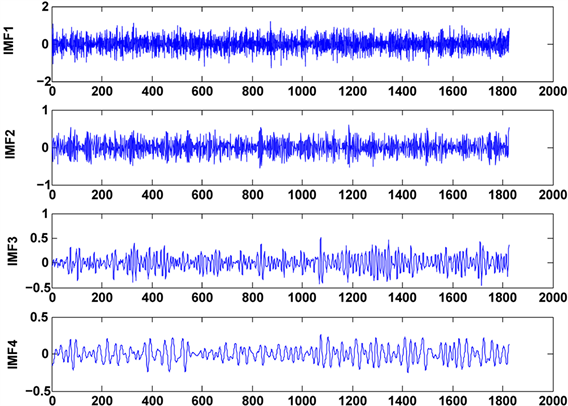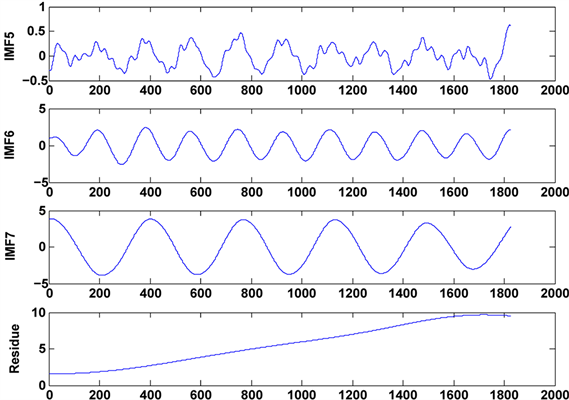Figure 4. Intrinsic mode component waveform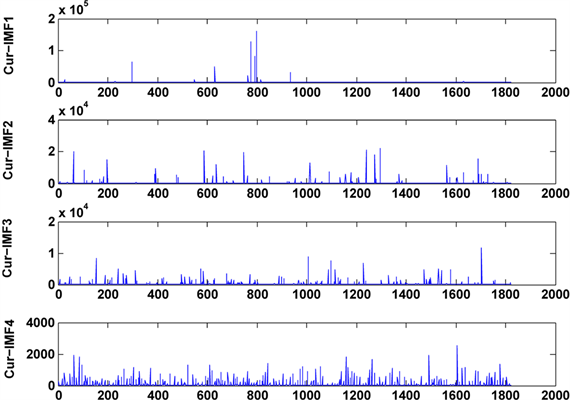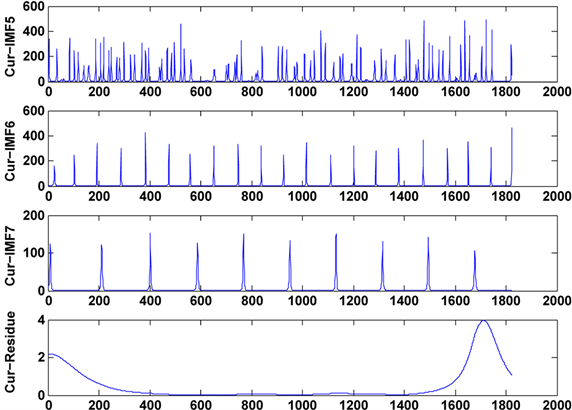Figure 5. Curvature curve waveform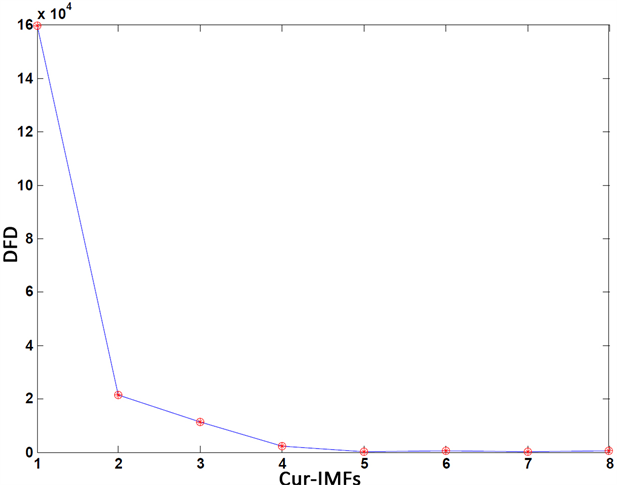Figure 6. Discrete Frechet distance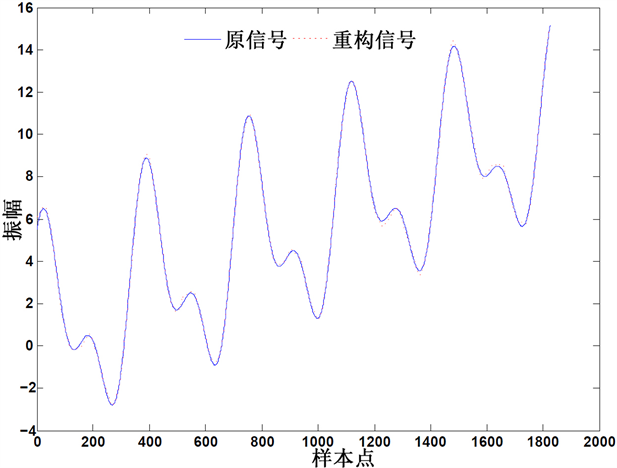Figure 7. Original signal and reconstructed signal waveform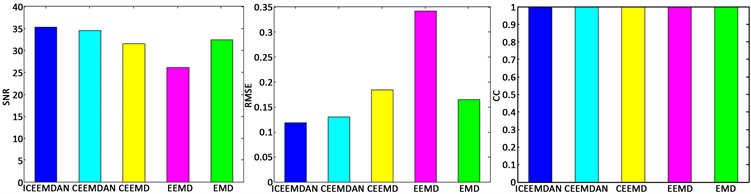Figure 8. Signal reconstruction effect of 5 kinds of methodsTable 1. Signal reconstruction effect of 5 kinds of methods

5. 结论

The Research of Denoising Method Based on Empirical Mode Decomposition Theory[J]. 应用数学进展, 2017, 06(07): 881-891. http://dx.doi.org/10.12677/AAM.2017.67106

1. 1. 李士心, 刘鲁源. 基于小波阈值去噪方法的研究[C]//中国仪器仪表学会. 中国仪器仪表学会第四届青年学术会议: 2002.

2. 2. Wang, L., Song, W., and Liu, P. (2016) Link the Remote Sensing Big Data to the image Features via Wavelet Transformation. Cluster Computing, 19, 793-810. https://doi.org/10.1007/s10586-016-0569-6

3. 3. Huang, N.E., Shen, Z., Long, S.R., Wu, M.C., Shih, H.H., Zheng, Q., Yen, N., Tung, C.C. and Liu, H.H. (1998) The Empirical Mode Decomposition and the Hilbert Spectrum for Non-Linear and Non-Stationary Time Series Analysis. Proceedings of the Royal Society of London A, 454, 903-995. https://doi.org/10.1098/rspa.1998.0193

4. 4. Wu, Z. and Huang, N.E. (2009) Ensemble Empirical Mode Decomposition:A Noise-Assisted Data Analysis Method. Advances in Adaptive Data Analysis, 1, 1-41. https://doi.org/10.1142/S1793536909000047

5. 5. Yeh, J.-R., Shieh, J.-S. and Huang, N.E. (2010) Complementary Ensemble Empirical Mode Decomposition: A Novel Noise Enhanced Data Analysis Method. Advances in Adaptive Data Analysis, 2, 135-156. https://doi.org/10.1142/S1793536910000422

6. 6. Torres, M.E., Colominas, M.A., Schlotthauer, G. and Flandrin, P. (2011) A Complete Ensemble Empirical Mode Decomposition with Adaptive Noise. In: 2011 IEEE International Conference on Acoustics, Speech and Signal Processing, Prague, 22-27 May 2011, 4144-4147. https://doi.org/10.1109/ICASSP.2011.5947265

7. 7. Colominas, M.A., Schlotthauer, G. and Torres, M.E. (2014) Improved Complete Ensemble EMD: A Suitable Tool for Biomedical Signal Processing. Biomedical Signal Processing and Control, 14, 19-29. https://doi.org/10.1016/j.bspc.2014.06.009

8. 8. Coolidge, J.L. (1952) The Unsatisfactory Story of Curvature. The American Mathematical Monthly, 59, 375-379. https://doi.org/10.2307/2306807

9. 9. Sriraghavendra, R., Karthik, K. and Bhattacharyya, C. (2007) Frechet Distance Based Approach for Searching Online Handwritten Documents. Proceeding ICDAR '07 Proceedings of the Ninth International Conference on Document Analysis and Recognition, Washington DC, 23-26 September 2007, 461-465.